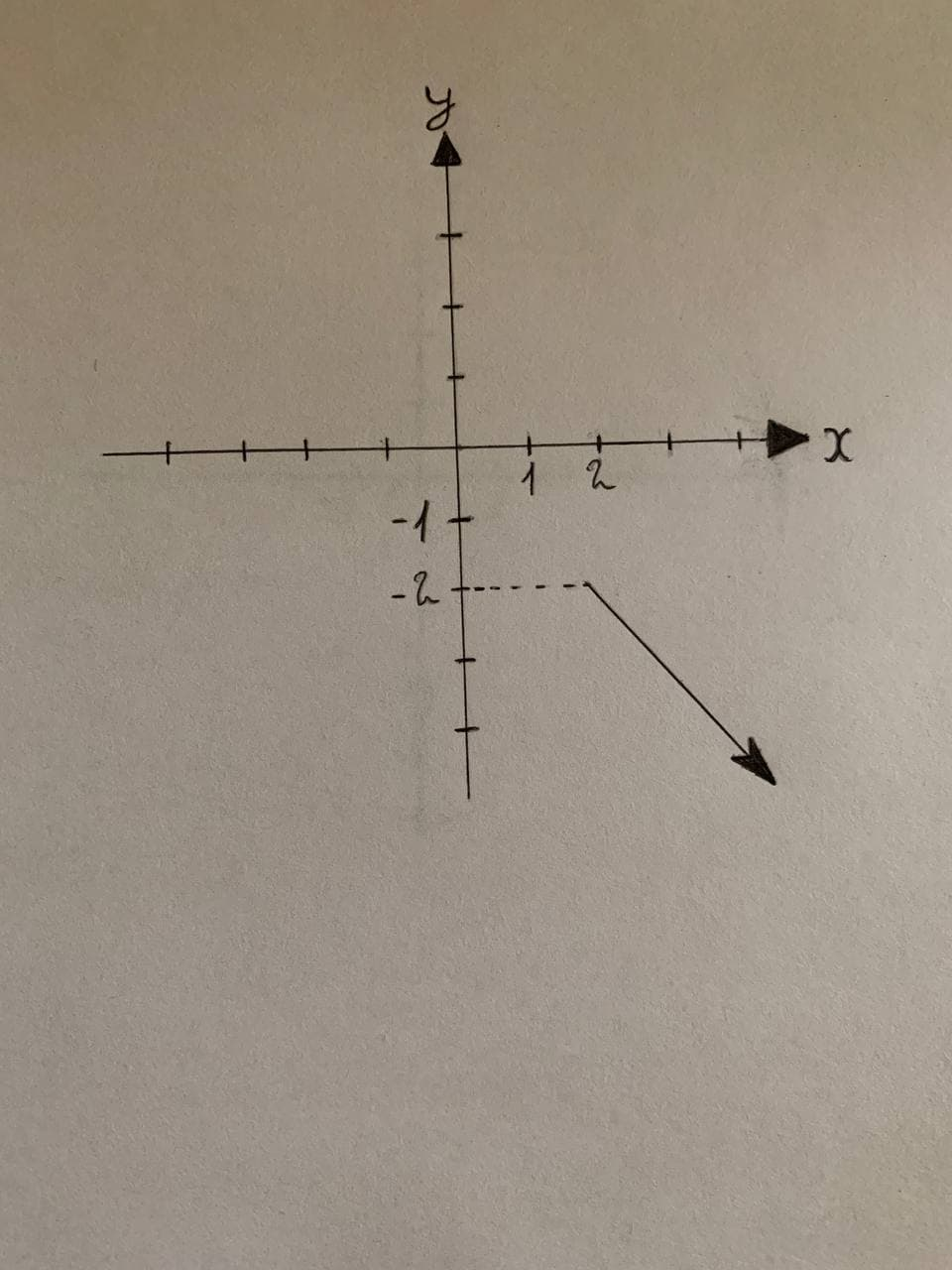Virginia Caron

2021-11-22

Graph the sets of points whose polar coordinates satisfy the given polar equation.
The given polar equation is written as follows:
$\theta =\frac{2\pi }{3},r\le -2$pendukke

Description:
Consider the polar coordinates as $P\left(r.\theta \right)$.
Here, r is the directed distance from origin Oto point Pand $\theta$ is the directed angle from initial ray to OP.
From the given equation, the range of r is from —2 to $—\propto$ and 0s fixed at $\frac{2\pi }{3}$. Therefore, the polar point traces a straight line at an angle of $\frac{2\pi }{3}$ from the initial ray (x-axis) and the line also satisfies the range of r.
From the analysis, draw the sets of points whose polar coordinates satisfy the given polar equation as shown in Figure 1.
$\theta =\frac{2\pi }{3},$
$r\le -2$Do you have a similar question?